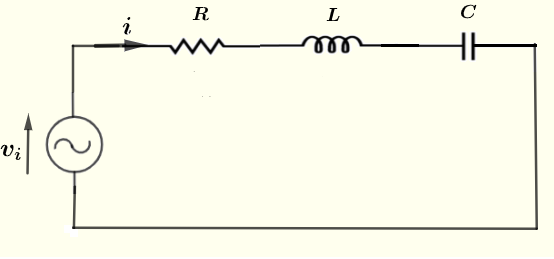# Resonant Series RLC Circuit Calculator

  

Calculators to calculate the characteristics of a resonant series RLC circuit are presented.Two calculators are included.
Calculator 1 may be used to calculate the resonanant, cutoff frequencies and the quality factor given the resistance $R$, the capacitance $C$ and the inductance $L$ included in the series RLC circuit as shown above.
Calculator 2 may be used solve the inverse problem. The cutoff frequencies and the resistance are given and the capaciatance, inductance and the quality factor are calculated.

## Formula of the Resonant and Cutoff Frequencies and the Quality Factor of an RLC Series Circuit

Several formula related to resonant series RLC circuit to be used in the calculators are reproduced here. The resonant frequency $\omega_r = \dfrac{1}{\sqrt {L C}}$ The cutoff frequencies
$\omega_{c1} = - \dfrac{R}{2 L} + \sqrt{ \left(\dfrac{R}{2 L}\right)^2 + \omega_r^2}$
$\omega_{c2} = \dfrac{R}{2 L} + \sqrt{ \left(\dfrac{R}{2 L}\right)^2 + \omega_r^2}$
Bandwidth $\Delta \omega = \omega_{c2} - \omega_{c1}$
The quality factor of the resonant circuit.
$Q = \dfrac{\omega_r}{\Delta \omega}$

## Use of the calculator 1 : Given R, L and C Calculate the Resonant, Cutoff Frequencies, Bandwidth and Quality Factor

Enter the resistance, the capacitance, the inductance and the frequency as positive real numbers with the given units then press "calculate".

 Resistance R = 300 mΩ Ω KΩ MΩ Capacitance C = 100 pF nF μF mF F Inductance L = 100 μH mH H

## Use of the calculator 2 : Given R and the Cutoff Frequencies, Calculate L, C and the Quality Factor Q

Enter the resistance and the cutoff frequencies as positive real numbers with the given units then press "calculate".

 Resistance R = 300 mΩ Ω KΩ MΩ Lower Cutoff Frequency $f_{c_1}$ = 1 GHz MHz kH Hz mHz Upper Cutoff Frequency $f_{c_2}$ = 100 GHz MHz kH Hz mHz